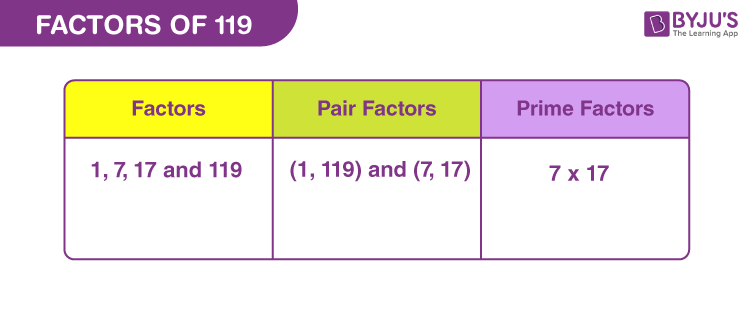# Factors of 119

In Maths, factors of 119 are the natural numbers by which the original number is completely divisible. If the multiplication of two numbers is 119 then the respective numbers are the factors. Suppose, the product of 1 and 119 is 119, therefore, both 1 and 119 are the factors. But, 119 is a composite number, therefore, it will have more than two factors.

The sum of seven consecutive prime numbers is equal to 119.

7 + 11 + 13 + 17 + 19 + 23 + 29 = 119

In this article, we will find the factors of 119 with easy and simple methods. Also, find the pair factors and prime factors of the number.## How to Find the Factors of 119?

To find the factors of 119 we have to divide it by the natural numbers that can evenly divide the original number. Hence, the remainder is equal to zero.

119 ÷ 1 = 119 [Remainder = 0]

119 ÷ 7 = 17 [Remainder = 0]

119 ÷ 17 = 7 [Remainder = 0]

119 ÷ 119 = 1 [Remainder = 0]

Thus, the factors of 119 are 1, 7, 17 and 119.

## Pair Factors of 119

The factors in pairs result in the original number, if multiplied together.

• 1 × 119 = 119
• 7 × 17 = 119

Therefore, the pair factors are (1, 119) and (7, 17).

• -1 × -119 = 119
• -7 × -17 = 119

Therefore, the negative pair factors are (-1, -119) and (-7, -17).

## Prime Factorisation of 119

The prime factors of 119 are the prime numbers that can exactly divide the original number. The prime factorisation of 119 will result in the required prime factors. In this method, we will be dividing the number 119 by the prime numbers, at every step of the resulting quotient, such that we are left only 1, at the end.

Step 1: Divide 119 by the smallest prime factor.

119 ÷ 7 = 17

Step 2: Now, 17 is a prime number and is divisible by itself.

17 ÷ 17 = 1

Therefore, the prime factors of 119 are 7 and 17.

 Prime factorisation of 119 = 7 x 17 Exponential Form = 71 x 171

## Solved Examples

Q.1: Find the sum of all the factors of 119.

Solution: The factors of 119 are 1, 7, 17 and 119 .

Sum = 1 + 7 + 17 + 119 = 144

Therefore, 144 is the required sum.

Q.2: What is the GCF of 119 and 126?

Answer: Let us first write the factors of both the numbers.

Factors of 119 → 1, 7, 17, 119

Factors of 126 → 1, 2, 3, 6, 7, 9, 14, 18, 21, 42, 63, 126

Therefore, the greatest common factor of 119 and 126 is 7.

Q.3: What are the common factors of 119 and 199?

119 → 1, 2, 43, 119

199 → 1, 199

Therefore, the common factor is 1.

### Practice Questions

1. Find the average of factors of 119.
2. Check if 119 and 179 are co-primes.
3. What is the GCF of 181 and 119?
4. By which numbers is 119 completely divisible?

## Frequently Asked Questions on Factors of 119

### What are the factors of 119?

There are a total of four factors of 119. These factors are 1, 7, 17 and 119.

### What two numbers make 119?

The product of two numbers that results in 119 is 7 x 17.

### Is 119 a prime number or composite number?

119 is a composite number. Any numbers having factors greater than two are composite.

### What is the largest factor of 119?

The greatest factor of 119 is 119 itself and the smallest factor is 1.

### Is 119 a perfect square?

119 is not a perfect square because the product of no such natural number results in 119.